# How Many NaCl Formula Units Does It Contain? express your answer using two significant figures

How many NaCl formula units does it contain? Express your answer using two significant figures.

A salt crystal has a mass of 0.16 mg .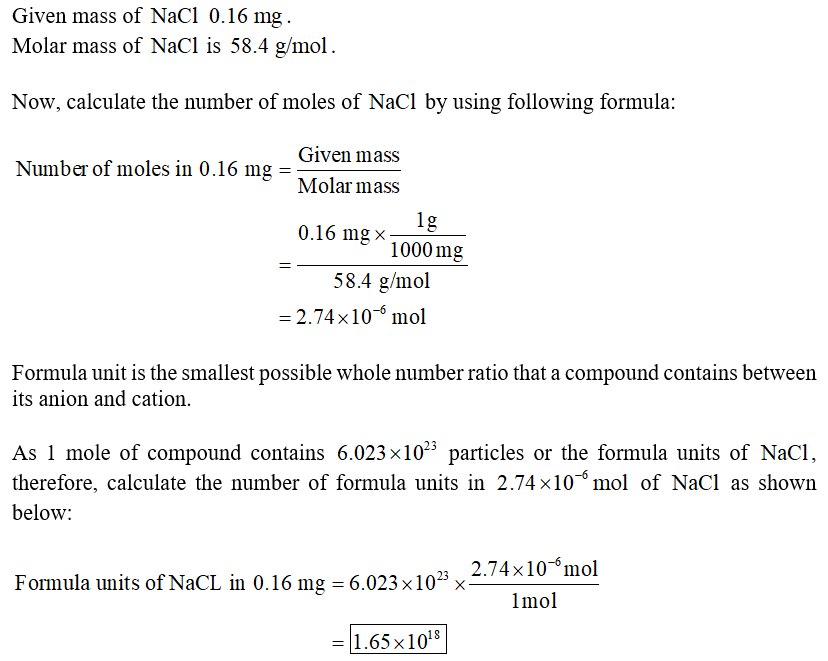Similar Homework Help Questions
• ### Step 2: Enter brief description of your problemA salt crystal has a mass of 0.12 mg. How many...

A salt crystal has a mass of 0.12 mg. How many NaCl formula units does it contain?

• ### What is its mass in mg? Express your answer using two significant figures. V Αφ ?...What is its mass in mg? Express your answer using two significant figures. V Αφ ? mg m = t A sugar crystal contains approximately 2.1x1017 (C12H22 O11) molecules. sucrose

• ### 15 in Express your answer to two significant figures and include the appropriate units Value Units...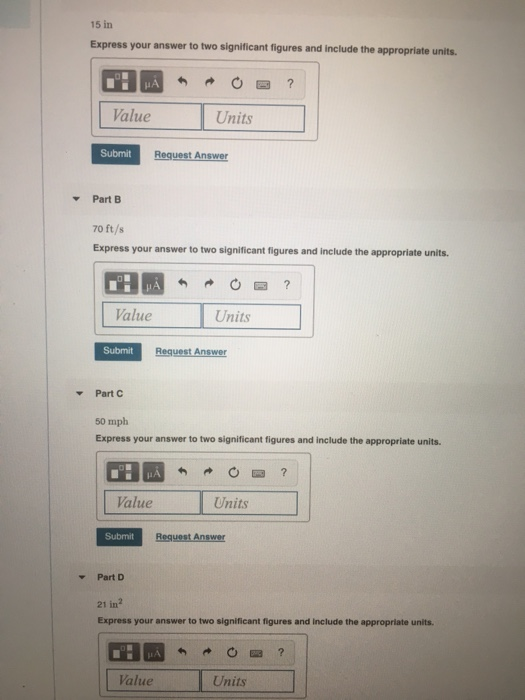15 in Express your answer to two significant figures and include the appropriate units Value Units Submit Request Answer Part B 70 ft/s Express your answer to two significant figures and include the appropriate units. alue Units Submit An Part 50 mph Express your answer to two significant figures and include the appropriate units. Value Units Submit Part D 21 in Express your answer to two significant figures and Include the appropriate units. Value Units

• ### chem

A salt crystal has a mass of .12 mg. How many NaCl formula units does it contain?

• ### How many moles of ibuprofen contain 1.51×10^23 atoms of carbon? Express your answer using three significant...

How many moles of ibuprofen contain 1.51×10^23 atoms of carbon? Express your answer using three significant figures.

• ### Find A . Express your answer to three significant figures with appropriate units. Find B. Express...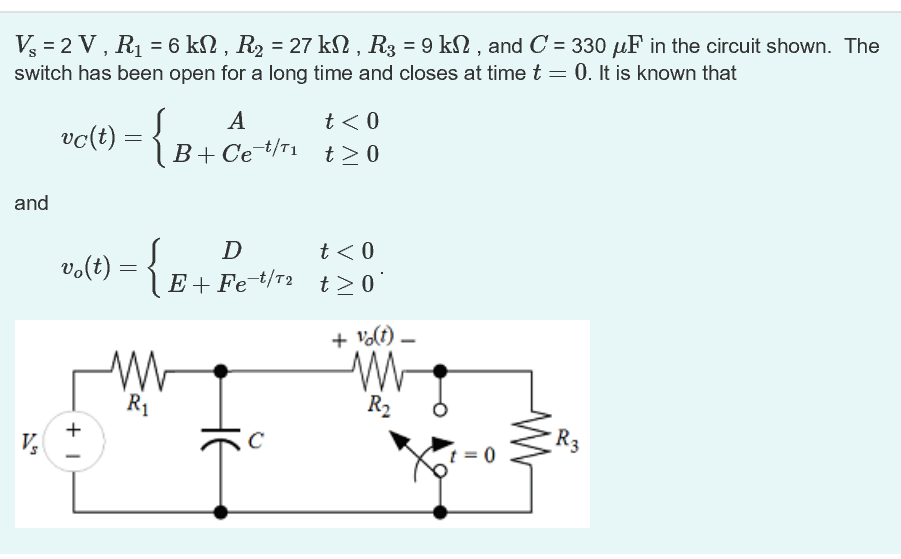Find A . Express your answer to three significant figures with appropriate units. Find B. Express your answer to three significant figures with appropriate units. Find C. Express your answer to three significant figures with appropriate units. Find ?1. Express your answer to three significant figures with appropriate units. Find D. Express your answer to three significant figures with appropriate units. Find E. Express your answer to three significant figures with appropriate units. Find F. Express your answer to three...

• ### What is the final temperature in C? Express your answer using two significant figures Review A...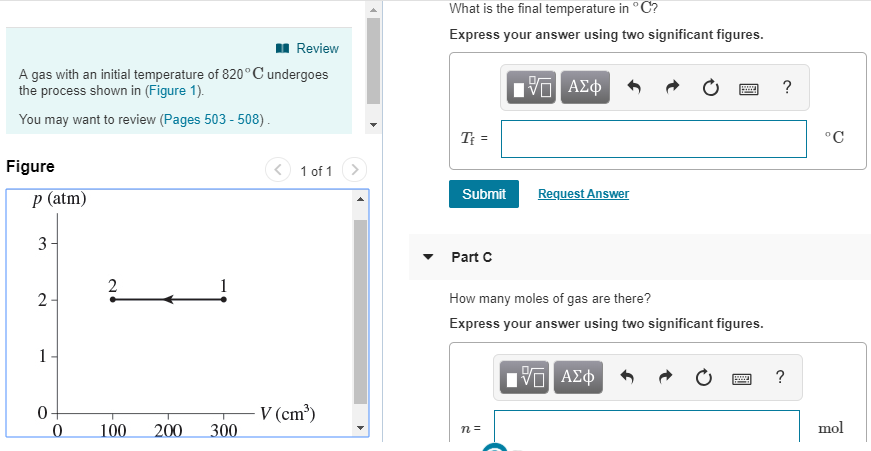What is the final temperature in C? Express your answer using two significant figures Review A gas with an initial temperature of 820 ° C undergoes the process shown in (Figure 1). You may want to review (Pages 503 - 508) Figure 1 of 1 Submit Request Answer p (atm) 3 Part C How many moles of gas are there? Express your answer using two significant figures 0 V (cm3) 100 2 300 mol

• ### How many atoms are there in 2.30 g Hg? Express your answer using appropriate units and...

How many atoms are there in 2.30 g Hg? Express your answer using appropriate units and significant figures.

• ### What is the formula weight of sodium carbonate? Express your answer to four significant figures and...

What is the formula weight of sodium carbonate? Express your answer to four significant figures and include the appropriate units.

• ### Express your answer using two significant figures. Review A point charge of-8.9 μC is at the...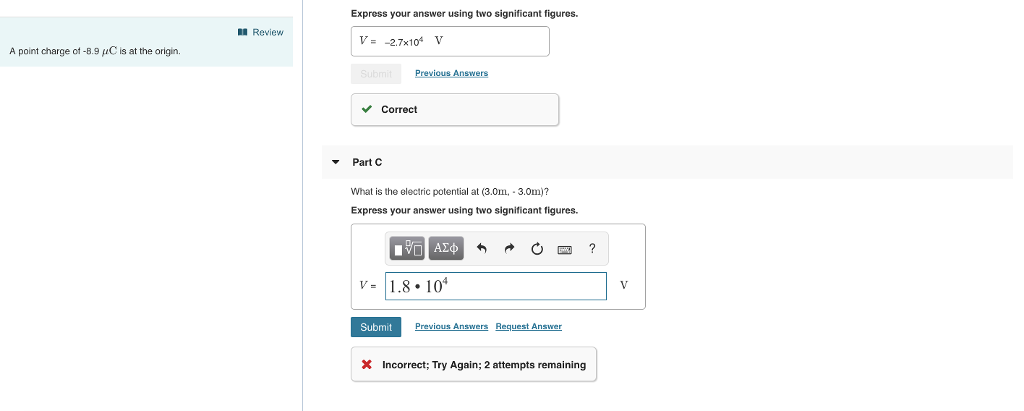Express your answer using two significant figures. Review A point charge of-8.9 μC is at the origin. Correct Part C What is the electric potential at (3.0m 3.0m)? Express your answer using two significant figures. ˇ- 11.8.104 Submit Previous Answers Request Answer Incorrect; Try Again; 2 attempts remaining

Need Online Homework Help?